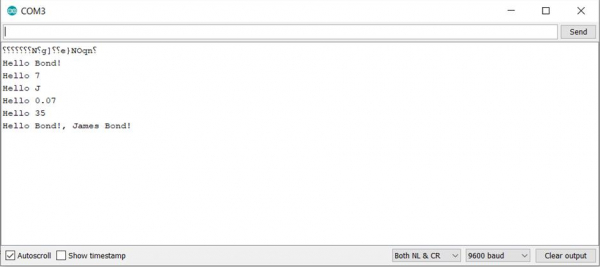# Concatenate strings in Arduino

String concatenation in Arduino is quite straightforward and also robust. You simply use the + operator to join strings. However, it doesn't end with joining two strings. You can concatenate characters, and even integers and floats to strings (Arduino converts the integers and floating-point numbers to string internally). Examples can be seen in the below code.

## Example

void setup() {
Serial.begin(9600);
Serial.println();
// put your setup code here, to run once:
String s1 = "Hello ";
String s2 = "Bond!";
String s3 = s1 + s2;
Serial.println(s3);
s3 = s1 + 7;
Serial.println(s3);
s3 = s1 + 'J';
Serial.println(s3);
s3 = s1 + 0.07;
Serial.println(s3);
s3 = s1 + millis();
Serial.println(s3);
s3 = s1 + s2 + ", James Bond!";
Serial.println(s3);
}
void loop() {
// put your main code here, to run repeatedly:
}

The output of this code is shown below −

## OutputAs you can see, we've successfully concatenated a string with another string, a character, an integer and even a floating-point number.

What this means that we can also concatenate the string with a function that outputs either a string, a character, an integer, or a floating-point number. We showed that by concatenating a string with the millis() function. Also, more than 2 strings can be concatenated in one statement, as shown by the last example in the above code.